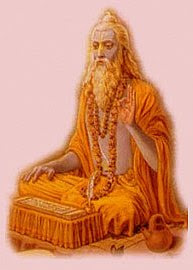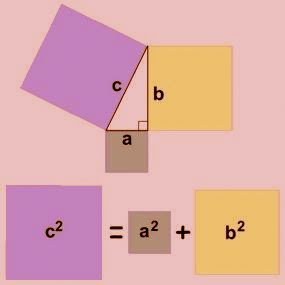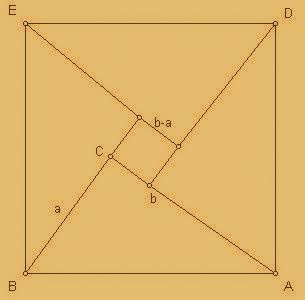## Saturday, January 17, 2009

### Pythagorus theorem was discovered by Indians and not by PythagorasYes, ancient Indians mathematicians discovered Pythagoras theorem! This might come as a surprise to many, but it's true that Pythagoras theorem was known much before Pythagoras and it was Indians who actually discovered it at least 1000 years before Pythagoras was born! It seems that Pythagoras stole this theorem from India and was given credit for it. It's one of the many examples of cases when Greek mathematicians/scientists took credit of various Indian discoveries/inventions and the original Indian contributers were forgotten.So which Indian mathematician discovered Pythagoras theorem originally? We now know that it was Baudhāyana who discovered the Pythagoras theorem. Baudhāyana listed Pythagoras theorem in his book called Baudhāyana Śulbasûtra (800 BCE). Incidentally, Baudhāyana Śulbasûtra is also one of the oldest books on advanced Mathematics. The actual shloka (verse) in Baudhāyana Śulbasûtra that describes Pythagoras theorem is given below -

Interestingly, Baudhāyana used a rope as an example in the above shloka which can be translated as - A rope stretched along the length of the diagonal produces an area which the vertical and horizontal sides make together. As you see, it becomes clear that this is perhaps the most intuitive way of understanding and visualizing Pythagoras theorem (and geometry in general) and Baudhāyana seems to have simplified the process of learning by encapsulating the mathematical result in a simple shloka in a layman's language (sanskrit was the language of choice back then).

Some people might say that this is not really an actual mathematical proof of Pythagorastheorem though and it is possible that Pythagoras provided that missing proof. But if we look in the same Śulbasûtra, we find that the proof of Pythagoras theorem has been provided by both Baudhāyana and Āpastamba in the Sulba Sutras! To elaborate, the shloka is to be translated as -

The diagonal of a rectangle produces by itself both (the areas) produced separately by its two sides.

The implications of the above statement are profound because it is directly translated into Pythagorean theorem (and graphically represented in the picutre on the left) and it becomes evident thatBaudhāyana proved Pythagoras theorem using area calculation and not geometry (as shown in the picture on left). Since most of the later proofs (presented by Euclid and others) are geometrical in nature, the Sulba Sutra's numerical proof was unfortunately ignored. As I mentioned before though, Baudhāyana was not the only Indian mathematician to have provided pythagorean triplets and proof. Āpastamba also provided the proof for Pythagoras theorem, which again is numerical in nature but again unfortunately this vital contribution has been ignored and Pythagoras was wrongly credited by Cicero and early Greek mathematicians for this theorem. For the sake of completeness, I should also mention that Baudhāyana also presented a geometrical proof using isosceles triangles so, to be more accurate, we attribute the geometrical proof to Baudhāyana and numerical (using number theory and area computation) proof to Āpastamba. Also, anotherancient Indian mathematician called Bhaskara later provided a unique geometrical proof as well (picuture illustrating Bhaskara's proof on right) which is known for the fact that it's truly generalized and works for all sorts of triangles and is not incongruent (not just isosceles as in some older proofs).

One thing that is really interesting is that Pythagoras was not credited for this theorem till atleast three centuries after! It was much later when Cicero and other greek philosophers/mathematicians/historians decided to tell the world that it was Pythagoras that came up with this theorem! How utterly ridiculous! In fact, later on many historians have tried to prove the relation between Pythagoras theorem and Pythagoras but have failed miserably. In fact, the only relation that historians have been able to trace it to is with Euclid, who again came many centuries after Pythagoras! This fact itself means that they just wanted to use some of their own to name this theorem after and discredit the much ancient Indian mathematicians without whose contribution it could've been impossible to create the very basis of algebra and geometry!

Many historians have also presented evidence for the fact that Pythagoras actually travelled to Egypt and then India and learned many important mathematical theories (including Pythagoras theorem) that western world didn't know of back then! So, it's very much possible that Pythagoras learned this theorem during his visit to India but hid his source of knowledge he went back to greece! This would also partially explain why greeks were so reserved in crediting Pythagoras with this theorem!

Looking at the implications of such fundamental laws like Pythagorean theorem that have been so grossly wrongly credited to greek mathematicians who have nothing to do with them, I wonder how many more other important ancient Indian discoveries and inventions have been wrongly credited so far!

To read about how Indians established potential prevention and cure of heart disease, diabetes, cancer and more, click - Hinduism Medical Discoveries _ Cure for cancer, heart disease, diabetes and more

To learn more about Lord Shiva, click - Shiva the Destroyer of Worlds and one of the Hindu Trinity

To learn more about Indian God Hanuman, click - Hanuman, the Rudravatar and the strongest but humble Indian god!

To learn the philosophy behind Hindu Trinity, click - Real meaning of Hindu Trinity - Brahma, Vishnu, Mahesh

To read about how Indians could've invented world's first super computer 3000 years back, click -
Antikythera mechanism - world's first computer - invented by Indians or Greeks?